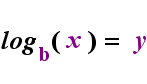Search

Logarithms: Introduction to
"The Relationship"
(page 1 of 3)

Sections: Introduction to logs, Simplifying log expressions, Common and natural logs

Logarithms are the "opposite" of exponentials, just as subtraction is the opposite of addition and division is the opposite of multiplication. Logs "undo" exponentials. Technically speaking, logs are the inverses of exponentials.

In practical terms, I have found it useful to think of logs in terms of The Relationship:

The Relationship
 y = bx ..............is equivalent to............... (means the exact same thing as) logb(y) = x

On the left-hand side above is the exponential statement "y = bx". On the right-hand side above, "logb(y) = x" is the equivalent logarithmic statement, which is pronounced "log-base-b of y equals x"; The value of the subscripted "b" is "the base of the logarithm", just as b is the base in the exponential expression "bx". And, just as the base b in an exponential is always positive and not equal to 1, so also the base b for a logarithm is always positive and not equal to 1. Whatever is inside the logarithm is called the "argument" of the log. Note that the base in both the exponential equation and the log equation (above) is "b", but that the x and y switch sides when you switch between the two equations.

 The Relationship AnimatedIf you can remember this relationship (that whatever had been the argument of the log becomes the "equals" and whatever had been the "equals" becomes the exponent in the exponential, and vice versa), then you shouldn't have too much trouble with logarithms.

(I coined the term "The Relationship" myself. You will not find it in your text, and your teachers and tutors will have no idea what you're talking about if you mention it to them. "The Relationship" is entirely non-standard terminology. Why do I use it anyway? Because it works.)

By the way: If you noticed that I switched the variables between the two boxes displaying "The Relationship", you've got a sharp eye. I did that on purpose, to stress that the point is not the variables themselves, but how they move.

• Convert "63 = 216" to the equivalent logarithmic expression.

To convert, the base (that is, the 6)remains the same, but the 3 and the 216 switch sides. This gives me:

• Convert "log4(1024) = 5" to the equivalent exponential expression.

To convert, the base (that is, the 4) remains the same, but the 1024 and the 5 switch sides. This gives me:

45 = 1024

Top  |  1 | 2 | 3  |  Return to Index  Next >>

 Cite this article as: Stapel, Elizabeth. "Logarithms: Introduction to 'The Relationship'." Purplemath. Available from     https://www.purplemath.com/modules/logs.htm. Accessed [Date] [Month] 2016

Study Skills Survey

Tutoring from Purplemath
Find a local math tutor